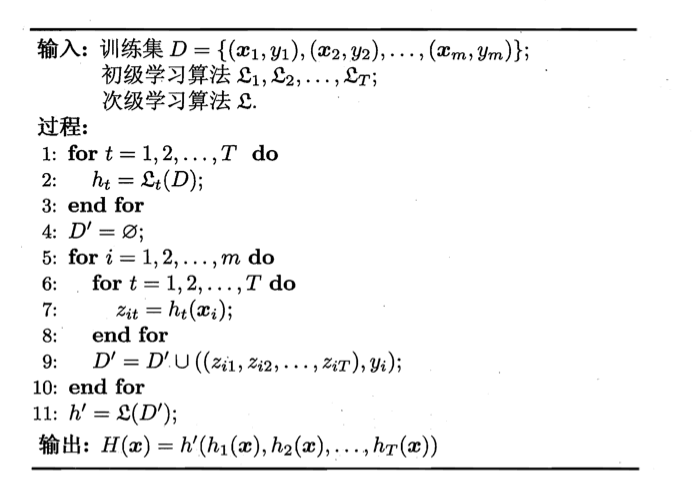# 【机器学习基础】第三十课：集成学习之结合策略

## 平均法（简单平均法、加权平均法），投票法（绝对多数投票法、相对多数投票法、加权投票法），学习法（Stacking）

Posted by x-jeff on November 24, 2021

【机器学习基础】系列博客为参考周志华老师的《机器学习》一书，自己所做的读书笔记。

# 2.平均法

👉简单平均法（simple averaging）

$H(x) = \frac{1}{T} \sum^T_{i=1} h_i (x)$

👉加权平均法（weighted averaging）

$H(x) = \sum^T_{i=1} w_i h_i(x)$

# 3.投票法

• 绝对多数投票法（majority voting）：即若某标记得票过半数，则预测为该标记；否则拒绝预测。
• 相对多数投票法（plurality voting）：即预测为得票最多的标记，若同时有多个标记获最高票，则从中随机选取一个。
• 加权投票法（weighted voting）：与加权平均法类似。

# 4.学习法

Stacking先从初始数据集训练出初级学习器，然后“生成”一个新数据集用于训练次级学习器。在这个新数据集中，初级学习器的输出被当作样例输入特征，而初始样本的标记仍被当作样例标记。Stacking的算法描述如下图所示：• 过程2：使用初级学习算法$\mathcal{L} _t$产生初级学习器$h_t$。
• 过程4：生成次级训练集。
• 过程11：在$\mathcal{D} ‘$上用次级学习算法$\mathcal{L}$产生次级学习器$h’$。

MLR是基于线性回归的分类器，它对每个类分别进行线性回归，属于该类的训练样例所对应的输出被置为1，其他类置为0；测试示例将被分给输出值最大的类。